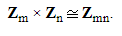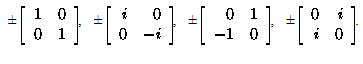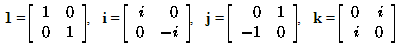# Groups, Subgroups, Cyclic Groups and Permutation Groups Notes | Study Algebra for IIT JAM Mathematics - Mathematics

## Mathematics: Groups, Subgroups, Cyclic Groups and Permutation Groups Notes | Study Algebra for IIT JAM Mathematics - Mathematics

The document Groups, Subgroups, Cyclic Groups and Permutation Groups Notes | Study Algebra for IIT JAM Mathematics - Mathematics is a part of the Mathematics Course Algebra for IIT JAM Mathematics.
All you need of Mathematics at this link: Mathematics

Groups, in general

3.1.3 Definition. A group (G,·) is a nonempty set G together with a binary operation · on G such that the following conditions hold:

(i) Closure: For all a,b  G the element a · b is a uniquely defined element of G.

(ii) Associativity: For all a,b,c  ∈ G, we have

a · (b · c) = (a · b) · c.

(iii) Identity: There exists an identity element e ∈ G such that

e · a = a and a · e = a

for all a ∈ G.

(iv) Inverses: For each a  G there exists an inverse element a-1 ∈ G such that

a · a-1 = e and a-1 · a = e.

We will usually simply write ab for the product a · b.

3.1.6. Proposition. (Cancellation Property for Groups) Let G be a group, and let a,b,c ∈ G.

(a) If ab=ac, then b=c.

(b) If ac=bc, then a=b.

3.1.8. Definition. A group G is said to be abelian if ab=ba for all a,b ∈ G.

3.1.9. Definition. A group G is said to be a finite group if the set G has a finite number of elements. In this case, the number of elements is called the order of G, denoted by |G|.

3.2.7. Definition. Let a be an element of the group G. If there exists a positive integer n such that an = e, then a is said to have finite order, and the smallest such positive integer is called the order of a, denoted by o(a).

If there does not exist a positive integer n such that an = e, then a is said to have infinite order.

3.2.1. Definition. Let G be a group, and let H be a subset of G. Then H is called a subgroup of G if H is itself a group, under the operation induced by G.

3.2.2. Proposition. Let G be a group with identity element e, and let H be a subset of G. Then H is a subgroup of G if and only if the following conditions hold:

(i) ab ∈ H for all a,b ∈ H;
(ii) e ∈ H;
(iii) a-1 ∈ H for all a ∈ H.

3.2.10. Theorem. (Lagrange) If H is a subgroup of the finite group G, then the order of H is a divisor of the order of G.

3.2.11. Corollary. Let G be a finite group of order n.

(a) For any a ∈ G, o(a) is a divisor of n.

(b) For any a ∈ G, an = e.

Example 3.2.12. (Euler's theorem) Let G be the multiplicative group of congruence classes modulo n. The order of G is given by (n), and so by Corollary 3.2.11, raising any congruence class to the power (n) must give the identity element.

3.2.12. Corollary. Any group of prime order is cyclic.

3.4.1. Definition. Let G1 and G2 be groups, and let θ : G1 -> G2 be a function. Then  is said to be a group isomorphism if

(i) θ is one-to-one and onto and

(ii) θ(ab) = θ(a) θ(b) for all a,b ∈ G1.

In this case, G1 is said to be isomorphic to G2, and this is denoted by G1 ≌ G2.

3.4.3. Proposition. Let θ : G1 -> G2 be an isomorphism of groups.

(a) If a has order n in G1, then θ(a) has order n in G2.

(b) If G1 is abelian, then so is G2.

(c) If G1 is cyclic, then so is G2.

Cyclic groups

3.2.5 Definition. Let G be a group, and let a be any element of G. The set is called the cyclic subgroup generated by a.

<a> = {x ∈ G | x = an for some n ∈ Z}

The group G is called a cyclic group if there exists an element a  G such that G=<a>. In this case a is called a generator of G.

3.2.6 Proposition. Let G be a group, and let a ∈ G.

(a) The set <a> is a subgroup of G.

(b) If K is any subgroup of G such that a  K, then <a>  K.

3.2.8. Proposition. Let a be an element of the group G.

(a) If a has infinite order, and ak = am for integers k,m, them k=m.

(b) If a has finite order and k is any integer, then ak = e if and only if o(a) | k.

(c) If a has finite order o(a)=n, then for all integers k, m, we have

ak = am if and only if k ≡ m (mod n).

Furthermore, |<a>|=o(a).

Corollaries to Lagrange's Theorem (restated):

(a) For any a ∈ G, o(a) is a divisor of |G|.

(b) For any a ∈ G, an = e, for n = |G|.

(c) Any group of prime order is cyclic.

3.5.1. Theorem. Every subgroup of a cyclic group is cyclic.

3.5.2 Theorem. Let G cyclic group.

(a) If G is infinite, then G ≌ Z.

(b) If |G| = n, then G ≌ Zn.

3.5.3. Proposition. Let G = <a> be a cyclic group with |G| = n.

(a) If m  Z, then <am> = <ad>, where d=gcd(m,n), and am has order n/d.

(b) The element ak generates G if and only if gcd(k,n)=1.

(c) The subgroups of G are in one-to-one correspondence with the positive divisors of n.

(d) If m and k are divisors of n, then <am <ak> if and only if k | m.

3.5.6. Definition. Let G be a group. If there exists a positive integer N such that aN=e for all a ∈ G, then the smallest such positive integer is called the exponent of G.

3.5.7. Lemma. Let G be a group, and let a,b ∈ G be elements such that ab = ba. If the orders of a and b are relatively prime, then o(ab) = o(a)o(b).

3.5.8. Proposition. Let G be a finite abelian group.

(a) The exponent of G is equal to the order of any element of G of maximal order.

(b) The group G is cyclic if and only if its exponent is equal to its order.

Permutation groups

3.1.4. Definition. The set of all permutations of a set S is denoted by Sym(S).

The set of all permutations of the set {1,2,...,n} is denoted by Sn.

3.1.5. Proposition. If S is any nonempty set, then Sym(S) is a group under the operation of composition of functions.

2.3.5. Theorem. Every permutation in Sn can be written as a product of disjoint cycles. The cycles that appear in the product are unique.

2.3.8 Proposition. If a permutation in Sn is written as a product of disjoint cycles, then its order is the least common multiple of the lengths of its cycles.

3.6.1. Definition. Any subgroup of the symmetric group Sym(S) on a set S is called a permutation group or group of permutations.

3.6.2. Theorem. (Cayley) Every group is isomorphic to a permutation group.

3.6.3. Definition. Let n > 2 be an integer. The group of rigid motions of a regular n-gon is called the nth dihedral group, denoted by Dn.

We can describe the nth dihedral group as

Dn = {ak, akb | 0 < k < n},

subject to the relations o(a) = n, o(b) = 2, and ba = a-1b.

2.3.11. Theorem. If a permutation is written as a product of transpositions in two ways, then the number of transpositions is either even in both cases or odd in both cases.

2.3.12. Definition. A permutation is called even if it can be written as a product of an even number of transpositions, and odd if it can be written as a product of an odd number of transpositions.

3.6.4. Proposition. The set of all even permutations of Sn is a subgroup of Sn.

3.6.5. Definition. The set of all even permutations of Sn is called the alternating group on n elements, and will be denoted by An.

Other examples

Example 3.1.4. (Group of units modulo n) Let n be a positive integer. The set of units modulo n, denoted by Zn×, is an abelian group under multiplication of congruence classes. Its order is given by the value φ(n) of Euler's phi-function.

3.1.10. Definition. The set of all invertible n × n matrices with entries in R is called the general linear group of degree n over the real numbers, and is denoted by GLn(R).

3.1.11. Proposition. The set GLn(R) forms a group under matrix multiplication.

3.3.3. Definition. Let G1 and G2 be groups. The set of all ordered pairs (x1,x2) such that x1 ∈ G1 and x2 ∈ G2 is called the direct product of G1 and G2, denoted by G1 × G2.

3.3.4. Proposition. Let G1 and G2 be groups.

(a) The direct product G1 × G2 is a group under the multiplication defined for all

(a1,a2), (b1,b2) ∈ G1 × G2 by

(a1,a2) (b1,b2) = (a1b1,a2b2).

(b) If the elements a1 ∈ G1 and a2 ∈ G2 have orders n and m, respectively, then in G1 × G2 the element (a1,a2) has order lcm[n,m].

3.3.5. Definition. Let F be a set with two binary operations + and · with respective identity elements 0 and 1, where 1 is distinct from 0. Then F is called a field if

(i) the set of all elements of F is an abelian group under +;

(ii) the set of all nonzero elements of F is an abelian group under ·;

(iii) a · (b+c) = a · b + a · c for all a,b,c in F.

3.3.6. Definition. Let F be a field. The set of all invertible n × n matrices with entries in F is called the general linear group of degree n over F, and is denoted by GLn(F).

3.3.7. Proposition. Let F be a field. Then GLn(F) is a group under matrix multiplication.

3.4.5. Proposition. If m,n are positive integers such that gcd(m,n)=1, thenExample. 3.3.7. (Quaternion group)
Consider the following set of invertible 2 × 2 matrices with entries in the field of complex numbers.If we letthen we have the identities

i2 = j2 = k2 = -1;

ij = k, jk = i, ki = j;

ji = -k, kj = -i, ik = -j.

These elements form a nonabelian group Q of order 8 called the quaternion group, or group of quaternion units.

The document Groups, Subgroups, Cyclic Groups and Permutation Groups Notes | Study Algebra for IIT JAM Mathematics - Mathematics is a part of the Mathematics Course Algebra for IIT JAM Mathematics.
All you need of Mathematics at this link: MathematicsUse Code STAYHOME200 and get INR 200 additional OFF

## Algebra for IIT JAM Mathematics

182 videos|58 docs

Track your progress, build streaks, highlight & save important lessons and more!

,

,

,

,

,

,

,

,

,

,

,

,

,

,

,

,

,

,

,

,

,

,

,

,

,

,

,

;